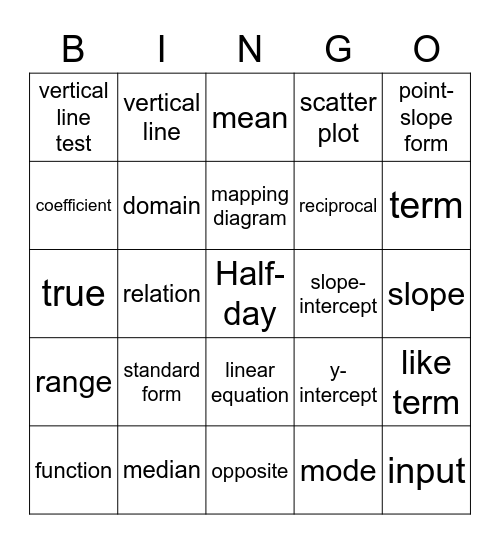# Algebra 2This bingo card has 39 words: matrix element, matrix, absolute value, domain, function, linear equation, mapping diagram, parent function, point-slope form, range, relation, scatter plot, Half-day, slope, slope-intercept, standard form, vertex, vertical line test, x-intercept, y-intercept, coefficient, opposite, reciprocal, term, variable, graph, input, output, horizontal line, vertical line, perpendicular line, parallel, mean, median, mode, constant, like term, true and false.

## Play Online